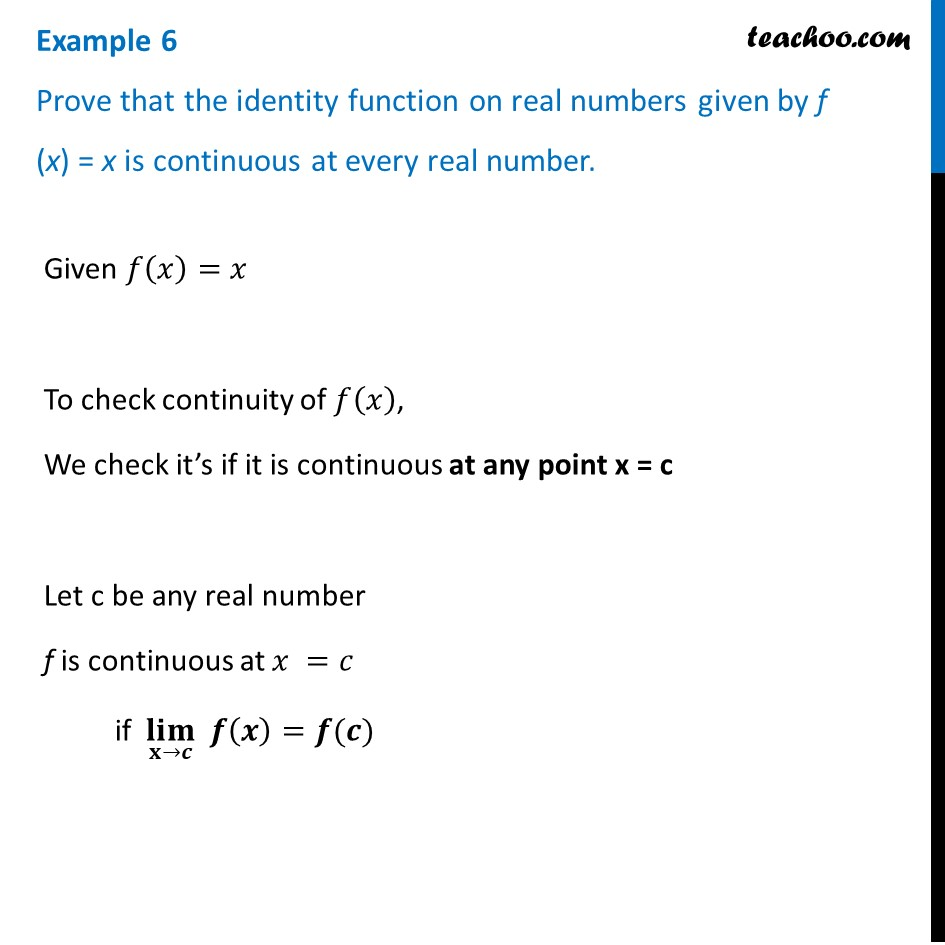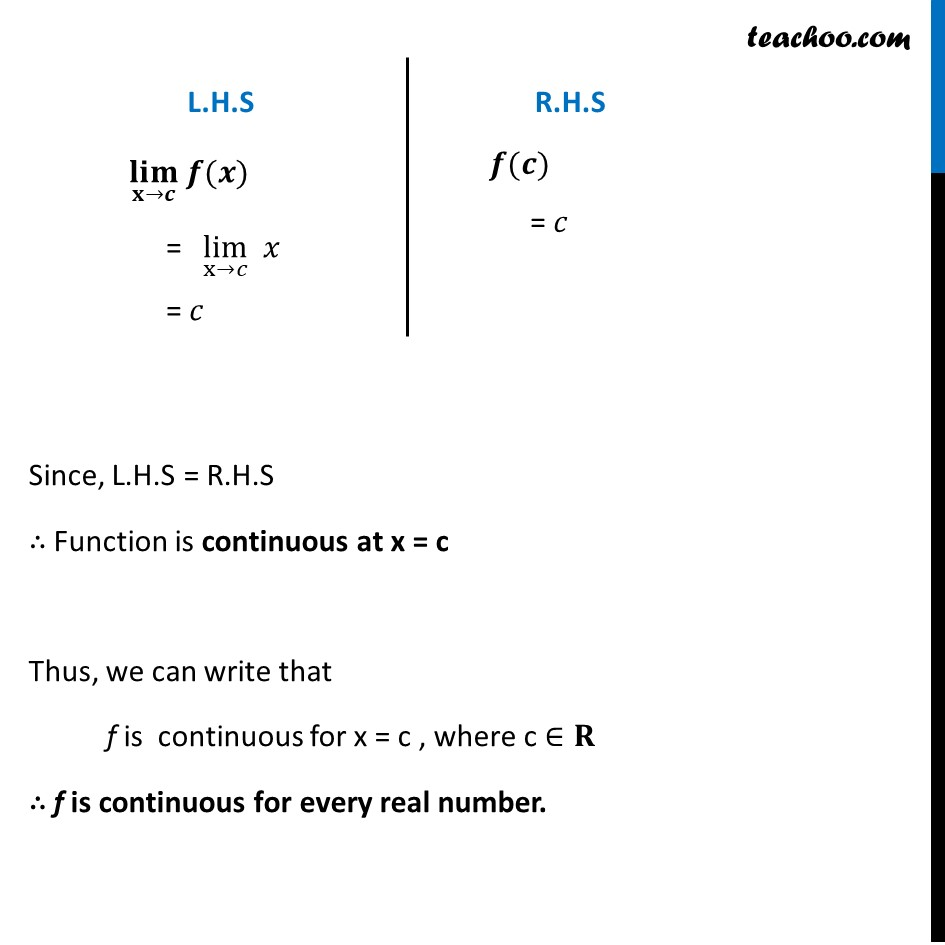Examples

Chapter 5 Class 12 Continuity and Differentiability
Serial order wiseLearn in your speed, with individual attention - Teachoo Maths 1-on-1 Class

### Transcript

Example 6 Prove that the identity function on real numbers given by f (x) = x is continuous at every real number.Given 𝑓(𝑥)=𝑥 To check continuity of 𝑓(𝑥), We check it’s if it is continuous at any point x = c Let c be any real number f is continuous at 𝑥 =𝑐 if (𝐥𝐢𝐦)┬(𝐱→𝒄) 𝒇(𝒙)=𝒇(𝒄) L.H.S (𝐥𝐢𝐦)┬(𝐱→𝒄) 𝒇(𝒙) "= " lim┬(x→𝑐) " " 𝑥 = 𝑐 R.H.S 𝒇(𝒄) = 𝑐 Since, L.H.S = R.H.S ∴ Function is continuous at x = c Thus, we can write that f is continuous for x = c , where c ∈𝐑 ∴ f is continuous for every real number.